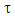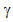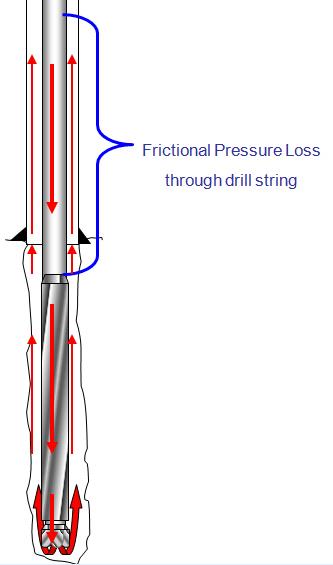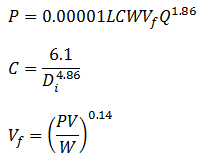## Power Law Constants (n and K) Calculation

Many types of drilling mud are classified as non-Newtonian fluid which is the behavior between Newtonian fluid model and Bingham Plastic model. The relationship between shear rate and shear stress is defined by the power law model shown below:Where= shear rate

K = consistency factor= shear rate

n = flow behavior index

## Pressure Losses Through Drill String CalculationTo determine pressure drop while the drilling mud is passing through a circular pipe (drill pipe and drill collar), you can calculate pressure loss with the following equation:Assumptions:

Turbulent viscosity = 3 cp

Friction factor = 0.378(Q/D)-0.14

The first equation has a coefficient (C) to take into account the friction and the geometry of the bore ID.

Where:

P = pressure loss, psi

L = length of pipe, ft

W = mud density, ppg

Vf = viscosity correction factor

PV = plastic viscosity, centipoises

Q = flow rate, gpm

Di = inside diameter of pipe, inch

C = general coefficient for internal diameter of pipe